How Cheenta works to ensure student success?
Explore the Back-Story

# Test of Mathematics Solution Subjective 73 - Coefficients of a QuadraticThis is a Test of Mathematics Solution Subjective 73 (from ISI Entrance). The book, Test of Mathematics at 10+2 Level is Published by East West Press. This problem book is indispensable for the preparation of I.S.I. B.Stat and B.Math Entrance.

Also visit: I.S.I. & C.M.I. Entrance Course of Cheenta

## Problem

Consider the equation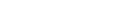, where G and H are complex numbers. Suppose that this equation has a pair of complex conjugate roots. Show that both G and H are real.

## Solution

Let three roots of the equationare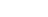[ Let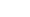are complex conjugates] .Now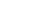= - H ... (i)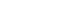= 0 ... (ii)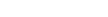= G ... (iii)

From (ii) we get= 0 [are complex conjugates so they are real]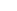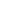= real Now as= real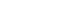=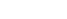=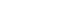=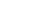... (iv)are complex conjugates so... (v) From (iv) & (v) we get=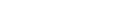G = real [from (iii)] Nowis real andis real so= realH = real.This is a Test of Mathematics Solution Subjective 73 (from ISI Entrance). The book, Test of Mathematics at 10+2 Level is Published by East West Press. This problem book is indispensable for the preparation of I.S.I. B.Stat and B.Math Entrance.

Also visit: I.S.I. & C.M.I. Entrance Course of Cheenta

## Problem

Consider the equation, where G and H are complex numbers. Suppose that this equation has a pair of complex conjugate roots. Show that both G and H are real.

## Solution

Let three roots of the equationare[ Letare complex conjugates] .Now= - H ... (i)= 0 ... (ii)= G ... (iii)

From (ii) we get= 0 [are complex conjugates so they are real]= real Now as= real===... (iv)are complex conjugates so... (v) From (iv) & (v) we get=G = real [from (iii)] Nowis real andis real so= realH = real.

### Knowledge Partner